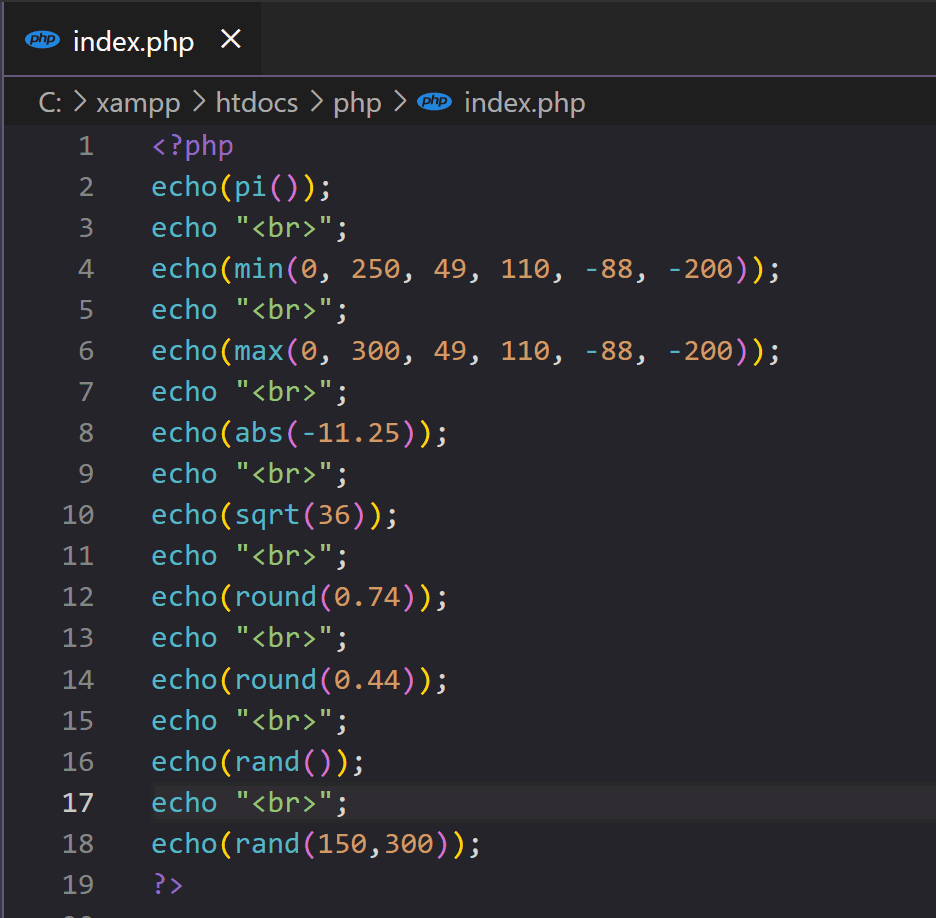# PHP Math Functions with Examples - PHP Math Operations

• PHP has a set of math functions that allows you to perform mathematical tasks on numbers.## PHP pi() Function

• The pi() function returns the value of PI:

## PHP min() and max() Functions

• The min() and max() functions can be used to find the lowest or highest value in a list of parameters:

## PHP abs() Function

• The abs() function returns the absolute (positive) value of a number

## PHP sqrt() Function

• The sqrt() function returns the square root of a number:

## PHP round() Function

• The round() function rounds a floating-point number to its nearest integer:

## Random Numbers

• The rand() function generates a random number:

## Output

• To control the random number values you have to give min and max values to show in the output